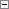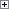Sets the dimensions of a variable that is to create a multi-dimensional variable (array).

Variables

##Remarks

Enter the dimensions as a semicolon-delimited list of integers, where each number defines the number of elements in that dimension. The maximum number of dimensions is 10 and the total number of elements in all dimensions is 1,000,000.

For example, to define an integer array with dimensions 3 x 3 x 4, start by creating an integer variable called A. In the Properties window, set the Dimensions property to a value of 3;3;4. To access the elements in the array, use either of the following:

• A
• A[2,3,2]

Indexes start at a value of 1 (not 0 as in some programming languages).

Array Functions